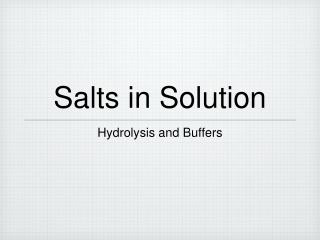DownloadDownload PresentationSalts in Solution

# Salts in Solution

Download Presentation## Salts in Solution

- - - - - - - - - - - - - - - - - - - - - - - - - - - E N D - - - - - - - - - - - - - - - - - - - - - - - - - - -
##### Presentation Transcript

1. Salts in Solution • Hydrolysis and Buffers

2. Introduction • Strong acids added to water produce a weak conjugate base. • HCl(g) + H2O(l) ➜ Cl-(aq) + H3O+(aq) strong acid weak base • Strong bases added to water produce a weak conjugate acid. • NaOH(s) ➜ Na+(aq) + OH-(aq) strong base weak acid

3. Introduction • Weak acids added to water produce a relatively strong conjugate base. • HNO2(aq) + H2O(l) ➜ NO2-(aq) + H3O+(aq) weak acid strong base • Weak bases added to water produce a relatively strong conjugate acid. • NH3(aq) + H2O(l) ➜ NH4+(aq) + OH-(aq) weak base strong acid

4. Salt Hydrolysis • When acids and bases become involved in neutralizations, they form salts and water. • HNO2(aq) + NaOH(aq) ➜ NaNO2(aq) + H2O(l) nitrous acid sodium hydroxide sodium nitrite water • HCl(aq) + NH3(aq) ➜ NH4Cl(aq) hydrochloric acid ammonium chloride ammonia • HCl(aq) + NaOH(aq) ➜ NaCl(aq) + H2O(l) hydrochloric acid sodium hydroxide sodium chloride water

5. Salt Hydrolysis • When the salts themselves are dissolved in water, they hydrolyze. • NaNO2(aq) ➜ Na+(aq) + NO2-(aq) sodium nitrite sodium nitrite • NH4Cl(aq) ➜ NH4+(aq) + Cl-(aq) ammonium chloride ammonium chloride • NaCl(aq) ➜ Na+(aq) + Cl-(aq) sodium chloride sodium chloride

6. Salt Hydrolysis • The ions that are weak conjugate acids and bases have no other effect on the solution.

7. Salt Hydrolysis • The ions that are relatively strong conjugate acids and bases have effects on the solution.

8. Salt Hydrolysis • Strong conjugate acids hydrolyze in solution, donate hydrogen ions, and lower the pH. • NH4+(aq) + H2O(l) ➜ NH3(aq) + H3O+(aq) • Strong conjugate bases hydrolyze in solution, accept hydrogen ions, and raise the pH. • CH3COO-(aq) + H2O(l) ➜ CH3COOH(aq) + OH-(aq)

9. Salt Hydrolysis • If we have a salt which results from the neutralization of a strong acid and a strong base • the resulting solution is neutral. • NaCl(aq) ➜ Na+(aq) + Cl-(aq) • Na+(aq) + H2O(l) ➜ no reaction • Cl-(aq) + H2O(l) ➜ no reaction

10. Salt Hydrolysis • If we have a salt which results from the neutralization of a strong acid and a weak base • the resulting solution is acidic. • NH4Cl(aq) ➜ NH4+(aq) + Cl-(aq) • NH4+(aq) + H2O(l) ➜ NH3(aq) + H3O+(aq) • Cl-(aq) + H2O(l) ➜ noreaction

11. Salt Hydrolysis • If we have a salt which results from the neutralization of a weak acid and a strong base • the resulting solution is basic. • NaClO(aq) ➜ Na+(aq) + ClO-(aq) • Na+(aq) + H2O(l) ➜ noreaction • ClO-(aq) + H2O(l) ➜ HClO(aq) + OH-(aq)

12. Salt Hydrolysis • If we have a salt which results from the neutralization of a weak acid and a weak base • the resulting solution may be acidic, basic, or neutral. • It depends on the relative strengths of the acid and base. • NH4ClO(aq) ➜ NH4+(aq) + ClO-(aq) • NH4+(aq) + H2O(l) ➜ NH3(aq) + H3O+(aq) • ClO-(aq) + H2O(l) ➜ HClO(aq) + OH-(aq)

13. Buffers • A buffer is a solution in which the pH remains relatively constant when small amounts of acid or base are added. • A buffer is prepared with a solution of • a weak acid and one of its salts • CH3COOH and NaCH3COO • a weak base and one of its salts • NH3 and NH4Cl

14. Buffers • Buffers are better able to resist pH changes than is pure water. • Add 10 mL of 0.1 M HCl to 50 mL of • pure water • pH goes from 7.00 to 1.78 (∆pH = 5.22) • acetic acid/acetate buffer • pH goes from 4.74 to 4.57 (∆pH = 0.18)

15. Buffers • The equilibrium set up between the acetic acid (CH3COOH) and its acetate salt (CH3COO-) allows the solution to absorb excess acid or base. • H3O+ + CH3COO- ⇄ CH3COOH + H2O • OH- + CH3COOH ⇄ CH3COO- + H2O • The concentrations of the acid and the salt act as reservoirs of neutralizing power.

16. Buffers • A buffer cannot control pH when too much acid or base is added. • The reservoirs of neutralizing power are used up. • When this happens, we exceed the buffering capacity of the system. • Our bodies keep blood at pH = 7.35-7.45 using • carbonic acid/hydrogen carbonate • dihydrogen phosphate/hydrogen phosphate